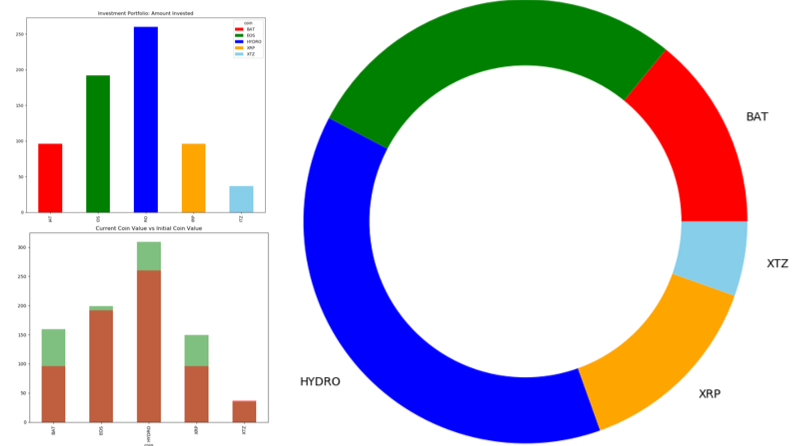# Using Pandas To Visualise Your Investment Portfolio

By Michael_Brown | Python For Crypto | 18 Feb 2020

Hi folks,

In this tutorial, I thought it would be a good idea to start using pandas to visualise our investment portfolio. This will allow us to see:

• The different coins we have invested in.
• The proportions each coin makes in our portfolio.
• The increase in each coin since we began investing.
• The total amount of money won or lost.

Having a way to visualise your portfolio will allow a quick and easy verification of which assets are performing and which are not, adding another weapon to your arsenal of financial analysis with Python.

To begin with, we'll import the necessary modules and create some mock data (you can simply copy and replace with your investment data):

import pandas as pd
import matplotlib.pyplot as plt

coin_dict = [
{'date': '01/02/2020', 'coin': 'BAT', 'amount_of_coins': 332.63294413, 'total_cost': 48.01},
{'date': '03/02/2020', 'coin': 'BAT', 'amount_of_coins': 332.63294413, 'total_cost': 48.01},
{'date': '05/02/2020', 'coin': 'XRP', 'amount_of_coins': 324.955628, 'total_cost': 48.01},
{'date': '07/02/2020', 'coin': 'XRP', 'amount_of_coins': 324.955628, 'total_cost': 48.01},
{'date': '09/02/2020', 'coin': 'XTZ', 'amount_of_coins': 15.615117, 'total_cost': 18.51},
{'date': '11/02/2020', 'coin': 'XTZ', 'amount_of_coins': 15.615117, 'total_cost': 18.51},
{'date': '13/02/2020', 'coin': 'EOS', 'amount_of_coins': 29.254878, 'total_cost': 96.00},
{'date': '15/02/2020', 'coin': 'EOS', 'amount_of_coins': 29.254878, 'total_cost': 96.00},
{'date': '12/02/2020', 'coin': 'HYDRO', 'amount_of_coins': 266666.666666, 'total_cost': 130},
{'date': '19/02/2020', 'coin': 'HYDRO', 'amount_of_coins': 266666.666666, 'total_cost': 130},
]

# Turn the list of dictionaries into a dataframe
coin_df = pd.DataFrame(coin_dict)

If we take a look at our coin_df dataframe: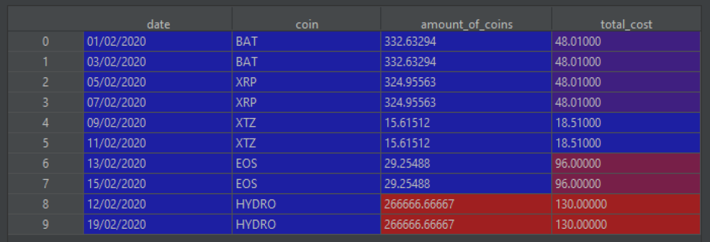Another column that would be good to have is the cost per coin, which will allow for a direct comparison between the initial investment cost and the current value of the coin. We can add this column using:

# Create a new columns for the cost per coin
coin_df['cost_per_coin'] = coin_df['total_cost'] / coin_df['amount_of_coins']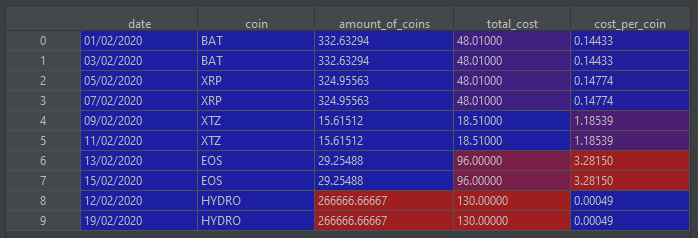With our dataframe containing all the information we need, we can create a new dataframe that is indexed by date, and has columns representing the total cost for all the coins listed in the 'coin' column:

total_cost = coin_df.pivot(index='date', columns='coin', values='total_cost')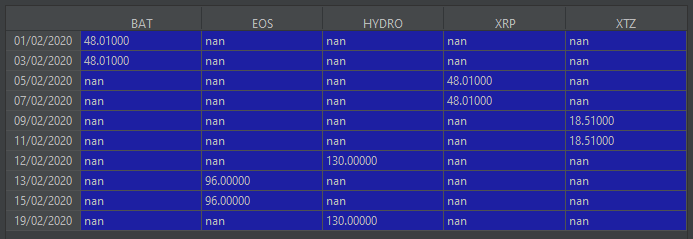We can quickly plot our investment portfolio:

total_cost.plot(kind='bar',
title='Investment Portfolio: Amount Invested',
color=['red', 'green', 'blue', 'orange', 'skyblue'])
plt.show()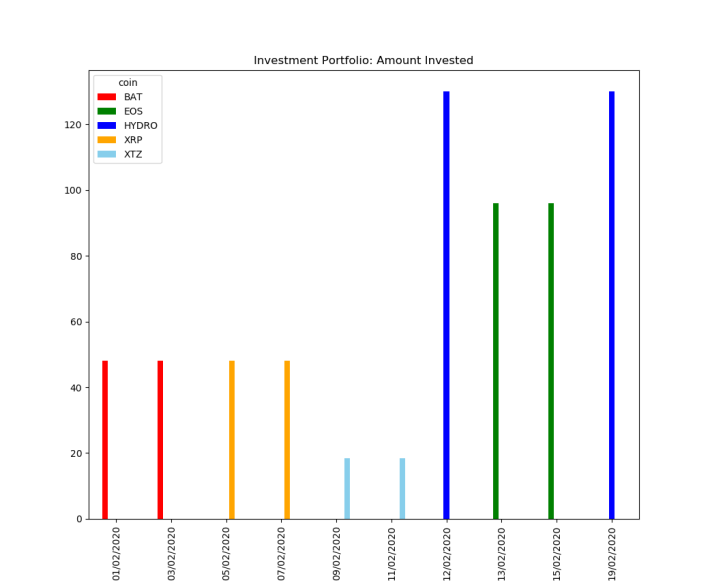Okay, that's not bad but it would be nice if we could group the coins together and get a total for each investment:

# Group the sum of coins for each different coin
total_investment = total_cost.sum()
total_investment.plot(kind='bar', color=['red', 'green', 'blue', 'orange', 'skyblue'])
plt.show()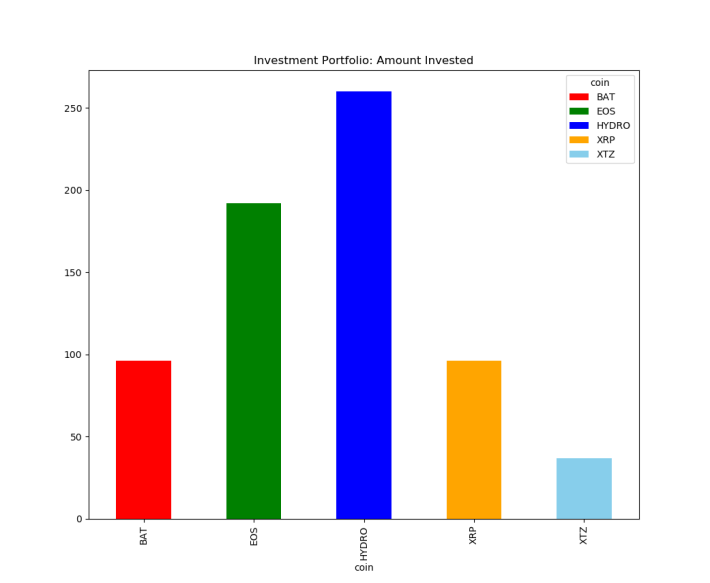Much better! We can immediately see where we've concentrated our funds in our investment portfolio. Another way to plot the above data could be by using a donut plot:

# create data for plot
# Series of coins and total amount spent
amount_data = total_cost.sum()
# Names of each coin (x values)
names = amount_data._index
# Total amount spent on each coin (y values)
size = amount_data.values

# Create a circle for the center of the plot
my_circle = plt.Circle((0, 0), 0.7, color='white')
# Give color names
plt.pie(size,
labels=names,
title='Investment Portfolio: Amount Invested',
colors=['red', 'green', 'blue', 'orange', 'skyblue'])
p=plt.gcf()
plt.show()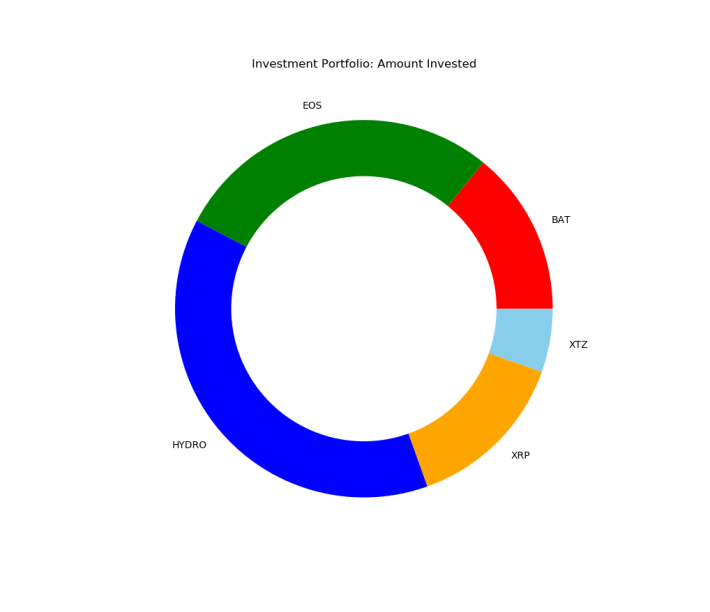I prefer this plot to the previous one, but there are many more plot types you could try if you wanted to (check out the matplotlib gallery for some inspiration)

One final aspect that would be beneficial to visualise is how our investment portfolio is performing today compared to when we first started. First, we'll need the current price of each coin, so let's create a dictionary to hold the current coin prices:

# Create a dictionary of the current price of all the coins we are interested in.
current_cost = {'BAT': 0.24, 'XRP': 0.23, 'XTZ': 1.14, 'EOS': 3.400, 'HYDRO': 0.00058}

Next, we'll pivot our coin_df dataframe but this time we want the total amount of coins in our portfolio for each coin we have:

# Create a series based on the total amount of coins we have in our possession
total_coins = coin_df.pivot(index='date', columns='coin', values='amount_of_coins').sum()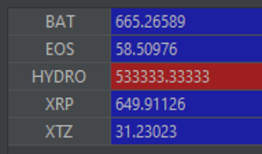The next step is to create two new series:

• Initial investment value
• Current investment value

# Create a list that will be the total number of each coin multiplied by the current value of that coin
current_value = [total_coins[coin_name] * current_cost[coin_name] for coin_name in total_coins._index]
# Turn the list into a series so we can plot it
current_value = pd.Series(current_value)
# Plot the two series, and use the alpha argument to increase the transparency and make it easier to see the two colours.
current_value.plot(kind='bar',
title='Current Coin Value vs Initial Coin Value',
alpha=0.5,
color='green')
total_investment.plot(kind='bar',
title='Current Coin Value vs Initial Coin Value',
alpha=0.5,
color='red')
plt.show()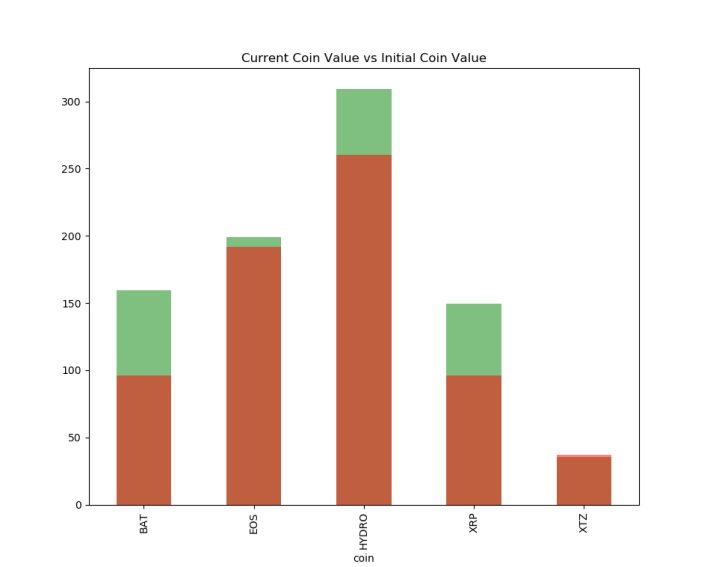Awesome, we are crusing it with our hypothetical investment portfolio! One final plot that would be nice to have is the total value of our initial investments compared to the current value of our investments:

# Now we can see whether our investments are performing well or not. Looking at the totals:
total_current_value = current_value.sum()
total_invested = total_investment.sum()
# Create the height of each bar based on the previous values
height = [total_invested, total_current_value]
# Create the positions for each value to be displayed at on the axis.
y_position = [0, 1]
# Assign the names of each value
bars = ('Initial Value', 'Value Today')
# Create bars
plt.bar(y_position, height)
# Create names on the x-axis
plt.xticks(y_position, bars)
# Set title
plt.title('Current Coin Value vs Initial Coin Value')
# Show graphic
plt.show()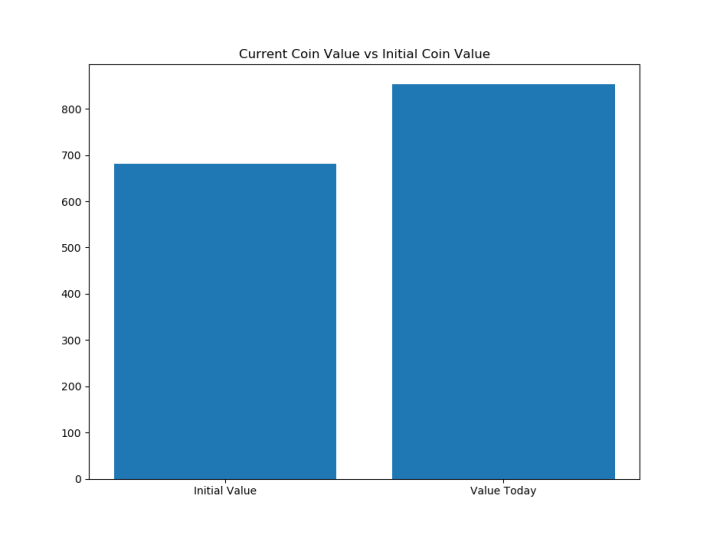With only a small amount of code, we've been able to create some effective visualisations of our investment portfolio. One possible next step would be to add a line of code to save each plot and then we could view all of the plots as and when we like. However, while this is a great means to see how our portfolio is performing, it feels a little static. It would be nice if we could have all (or more) of these visualisations combined onto a dashboard.

As such, I'm going to look into using a library called Dash to create an interactive app that will allow me to do the above visualisations all in one place. I've never used it before, but the great thing about Python is it is so intuitive and there are so many well-written tutorials out there I imagine it won't be too hard to pick up.

As always, I hope you've enjoyed this tutorial and the next time I see you I should be able to show off a fancy portfolio dashboard you can use to keep track of your portfolio :)Michael_Brown

Physics graduate, with a passion for programming in Python.Python For Crypto

I'm Michael, a Python developer and I'm documenting some useful libraries and techniques you can use for free to aid in your Crypto-analysis and Crypto-portfolio.Send a \$0.01 microtip in crypto to the author, and earn yourself as you read!

20% to author / 80% to me.
We pay the tips from our rewards pool.